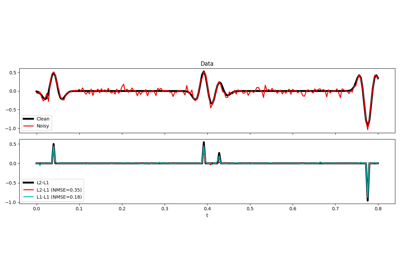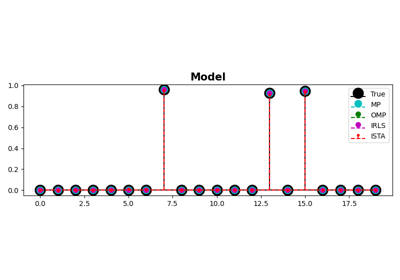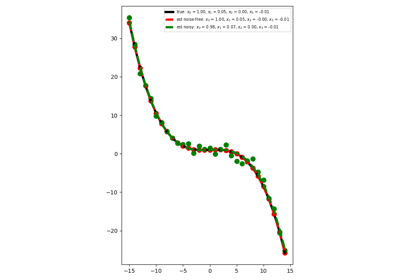# pylops.optimization.sparsity.irls#

pylops.optimization.sparsity.irls(Op, y, x0=None, nouter=10, threshR=False, epsR=1e-10, epsI=1e-10, tolIRLS=1e-10, warm=False, kind='data', show=False, itershow=(10, 10, 10), callback=None, **kwargs_solver)[source]#

Iteratively reweighted least squares.

Solve an optimization problem with $$L_1$$ cost function (data IRLS) or $$L_1$$ regularization term (model IRLS) given the operator Op and data y.

In the data IRLS, the cost function is minimized by iteratively solving a weighted least squares problem with the weight at iteration $$i$$ being based on the data residual at iteration $$i-1$$. This IRLS solver is robust to outliers since the L1 norm given less weight to large residuals than L2 norm does.

Similarly in the model IRLS, the weight at at iteration $$i$$ is based on the model at iteration $$i-1$$. This IRLS solver inverts for a sparse model vector.

Parameters
Oppylops.LinearOperator

Operator to invert

ynumpy.ndarray

Data

x0numpy.ndarray, optional

Initial guess

nouterint, optional

Number of outer iterations

threshRbool, optional

Apply thresholding in creation of weight (True) or damping (False)

epsRfloat, optional

Damping to be applied to residuals for weighting term

epsIfloat, optional

Tikhonov damping

tolIRLSfloat, optional

Tolerance. Stop outer iterations if difference between inverted model at subsequent iterations is smaller than tolIRLS

warmbool, optional

Warm start each inversion inner step with previous estimate (True) or not (False). This only applies to kind="data" and kind="datamodel"

kindstr, optional

Kind of solver (model, data or datamodel)

showbool, optional

Display logs

itershowtuple, optional

Display set log for the first N1 steps, last N2 steps, and every N3 steps in between where N1, N2, N3 are the three element of the list.

callbackcallable, optional

Function with signature (callback(x)) to call after each iteration where x is the current model vector

**kwargs_solver

Arbitrary keyword arguments for scipy.sparse.linalg.cg solver for data IRLS and scipy.sparse.linalg.lsqr solver for model IRLS when using numpy data(or pylops.optimization.solver.cg and pylops.optimization.solver.cgls when using cupy data)

Returns
xinvnumpy.ndarray

Inverted model

nouterint

Number of effective outer iterations

Notes

## Examples using pylops.optimization.sparsity.irls#L1-L1 IRLS

L1-L1 IRLSMP, OMP, ISTA and FISTA

MP, OMP, ISTA and FISTAPolynomial Regression

Polynomial Regression